• 关于图论中的导出子图作了一些比较容易懂得解释，我看网上有各种版本的甚至还有些错误的的，所以自己查询了一些资料对这些概念进行了图文解释。
• 针对经典计算的有向图k边导出子图生成算法时间复杂度较高问题,提出了一种在脱氧核糖核酸粘贴机上运行的子图生成算法.首先,以粘贴系统提供的标准生化元操作为算法使用的基本元算子,并使用元操作所产生的生化结果的...
• 关于图论中导出子图的概念 1、导出子图 A subgraph H is called an induced subgraph of X if for any a,b∈E(H)a,b \in E(H)a,b∈E(H) if and only if a,b∈E(X)a,b \in E(X)a,b∈E(X). 2、点导出子图 设S是V(G)的...
关于图论中导出子图的概念
1、导出子图
A subgraph H is called an induced subgraph of X if for any

a

,

b

∈

E

(

H

)

a,b \in E(H)

if and only if

a

,

b

∈

E

(

X

)

a,b \in E(X)

.
2、点导出子图
设S是V(G)的子集，以S为点集，以G的所有那些两端点都在S内的边组成边集，所得到的G的子图称为S在G中的导出子图，或更确切地，节点导出子图。（一个顶点子集以及两个端点都在这个子集中的所有边构成的子图）
例子：
在图1中，设G如图1(a)所示，取

V

1

=

{

a

,

b

,

c

}

{V_1} = \left\{ {a,b,c} \right\}

,则

V

1

{V_1}

的导出子图

G

(

V

1

)

G\left( {{V_1}} \right)

如图所示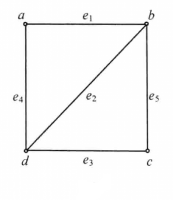导出子图

G

(

V

1

)

G\left( {{V_1}} \right)

：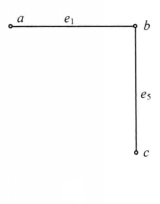3、边导出子图
一个边子集以及这些边的所有端点构成的子图.
例子：
在图1中，设G如图1(a)所示，取

E

1

=

{

e

1

,

e

3

}

{E_1} = \left\{ {e_1,e_3} \right\}

,则

E

1

{E_1}

的导出子图

G

(

E

1

)

G\left( {{E_1}} \right)

如图 所示导出子图

G

(

E

1

)

G\left( {{E_1}} \right)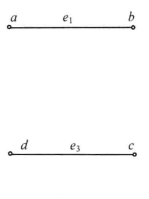展开全文• 给定一个无向图 G=(V,E)G=(V,E)G=(V,E) 和一个整数 kkk，试找到 GGG 的最大规模的导出子图 H=(U,F)H=(U,F)H=(U,F)，其中 HHH 中所有顶点的度大于或等于 kkk，或者说明不存在这样的子图。 归纳假设 假设在顶点数目...
问题描述
给定一个无向图

G

=

(

V

,

E

)

G=(V,E)

和一个整数

k

k

，试找到

G

G

的最大规模的导出子图

H

=

(

U

,

F

)

H=(U,F)

，其中

H

H

中所有顶点的度大于或等于

k

k

，或者说明不存在这样的子图。
归纳假设
假设在顶点数目小于

n

n

时，我们已知如何找到所有顶点的度大于或等于

k

k

的最大导出子图。
归纳证明
当

n

≤

k

n{\le}k

时，则所有度均小于

k

k

，不存在这样的图。当

n

=

k

+

1

n=k+1

时，则可以注意到所有度大于等于

k

k

的唯一方式是一个完全图。假定现在

n

n

是平凡的情况，即

n

>

k

+

1

n>k+1

。如果所有顶点的度大于或等于

k

k

，则整个图满足要求。否则，存在一个顶点

v

v

，其度小于

k

k

。显然在任何

G

G

的子图中，

v

v

的度都小于

k

k

，因此

v

v

不在任何满足原命题的子图中。所以把

v

v

及其相连的边删除，不影响原命题。当

v

v

被删除后，图中只有

n

−

1

n-1

个顶点。由归纳假设，可知如何对该问题求解。
由1，2得：对与任意无向图

G

=

(

V

,

E

)

G=(V,E)

和一个整数

k

k

，均能完成求解任务。
算法实现
def Max_Subgraph(G, k):
""" U (G的子图, None表示无这样的子图)

Args:
G (dict): 图的邻接表 e.g. {1:, 2:}表示节点为2的完全图
k (int): 度
"""
Du_More_Than = lambda G, n: all([len(G[v]) >= n for v in G])
Find_V = lambda G, n: [v for v in G if len(G[v]) < n]
while True:
if len(G) <= k:
return None
if len(G) == k + 1:
return G if Du_More_Than(G, k) else None
for v in Find_V(G, k):
for u in G[v]:
G[u].remove(v)
del G[v]

展开全文算法 python
• ## 子图，生成子图和导出子图

万次阅读 多人点赞 2016-10-08 23:46:43
设V1是V的一个非空子集，以V1为顶点集，以两端点均在V1中的边的全体为边集的子图称为G的导出子图，记作G[V1]。导出子图G[V\V1]记为G-V1，它是从G中删去V1中的顶点以及与这些顶点相关的边所得到的子图。若V1={v}，则...
所有的顶点和边都属于图G的图称为G的子图。含有G的所有顶点的子图称为G的生成子图。 设V1是V的一个非空子集，以V1为顶点集，以两端点均在V1中的边的全体为边集的子图称为G的导出子图，记作G[V1]。导出子图G[V\V1]记为G-V1，它是从G中删去V1中的顶点以及与这些顶点相关的边所得到的子图。若V1={v}，则把G-{v}简记为G-v， 称为主子图。在图1-13中，G1是G的生成子图，G2是G的导出子图，G3是G的主子图。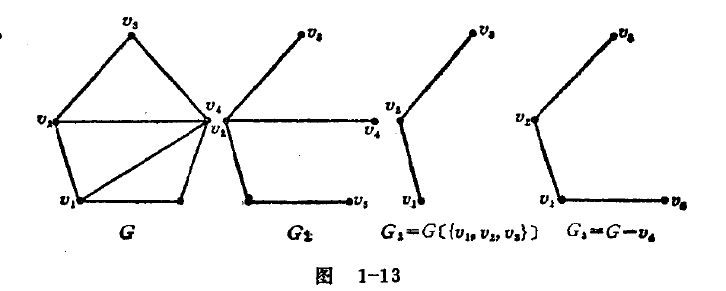展开全文• 首先给出一些定义。原图G用G = (V, E)表示，V是G中的所有顶点的集合；E是G中所有边的集合。 子图 定义：子图G’中所有的顶点和边均包含于原图G。即E’∈E，并且V’∈V。...定义：导出子图G’，V’∈V，但对...
首先给出一些定义。原图G用G = (V, E)表示，V是G中的所有顶点的集合；E是G中所有边的集合。
子图 定义：子图G’中所有的顶点和边均包含于原图G。即E’∈E，并且V’∈V。  生成子图（Spanning Subgraph） 定义：生成子图G’中顶点个数V’必须和原图G中V的数量相同，而E’∈E即可。  导出子图(Induced Subgraph) 定义：导出子图G’，V’∈V，但对于V’中任一顶点，只要在原图G中有对应边，那么就要出现在E’中。
举个栗子：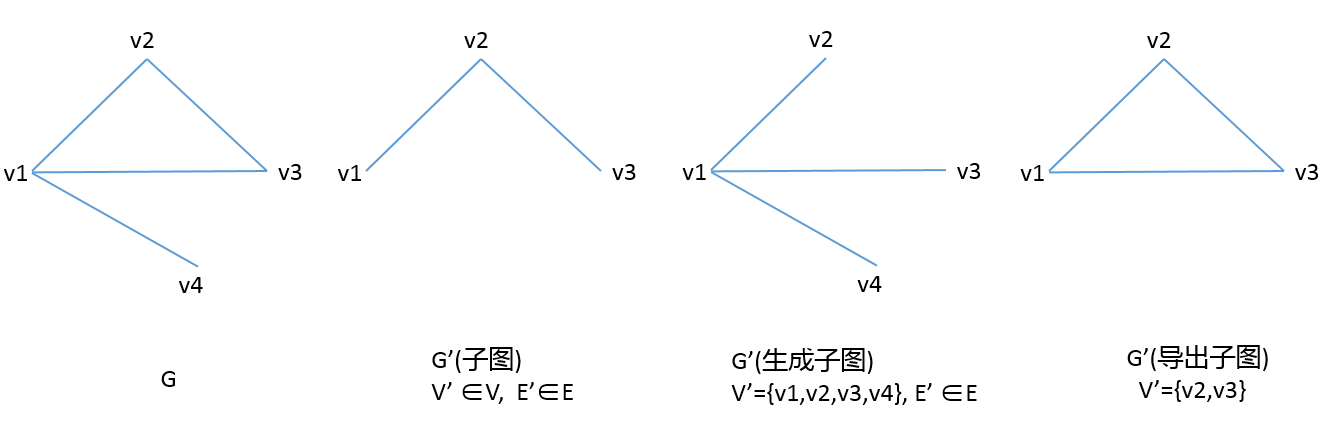展开全文•  设V1是V的一个非空子集，以V1为顶点集，以两端点均在V1中的边的全体为边集的子图称为G的导出子图，记作G[V1]。导出子图G[V\V1]记为G-V1，它是从G中删去V1中的顶点以及与这些顶点相关的边所得到的子图。若V1={v}，...ACM
• Erodos证明了对于一个图G，X(G)-ω(G)可以任意大。因此，对一般图而言，其色数不一定能找到一个与团...得到了如果图G是一个不含K1+P3和C4作为导出子图的图，那么当a(G)≥3时，X(G)=ω(G)；当α(G)=2时，X(G)n≤2ω(G)。
• Gyárfás曾猜想：对于每一个不含森林[F]作为导出子图的图[G]，存在整数函数[f(F,ω(G))]使得[χ(G)f(F,ω(G))]，其中[χ(G)]和[ω(G)]分别表示图的色数和团数。以强完美图定理为基础，通过对不含[3K1 K2]和[C4]...
• 题意简述：给定一张无向图和一个数，求一个最大的子图，使得删掉原图中其他点后，子图中每个点的点度都大等。要求输出子图大小和子图中的每个点，若无解输出，若存在多解输出任意一组解。 题目让我们求一个子图，最...
• 选择一个顶点导出子图，该子图的每个点点权为 该点连接的最小边权。 找一个这样的子图使得点权和最大，输出点权和。 思路： 因为是一个完全图，所以我们选择的点构成的图一定不包含权值为0的边。因为若包含了权值...
• ## 归纳法之导出子图

千次阅读 2010-07-08 09:59:00
• AtCoder Beginner Contest 173 F - ...f(L,R)f(L,R)f(L,R)：顶点集 V′={L,L+1,L+2,⋯ ,R}V'=\{L, L+1, L+2,\cdots,R\}V′={L,L+1,L+2,⋯,R}的导出子图（由 顶点集 V′V'V′ 和 两端点都在顶点集 V′V'V′中的边
• 求这张图上的所有导出子图中有多少棵树。 解题思路 条件可以转换为这些区间联通并且没有一个位置被333个区间同时覆盖。 那么如果一个包含关系出现在这个图里，那么被包含的那个一定是作为叶子的。所以可以按照左...dp
• 子图： 在子图中，E’<=E且V’<=V。 生成子图： 在生成子图中，E’<=E,且V’=V。 诱导子图： 对于V′的所有顶点,只要在G中有连边,这个边就在G′出现.也说G′是G的由V′诱导出的子图.记为G′＝G[V′]。 团：...社交网络 数据结构
• 2.点导出子图与边导出子图导出子图导出子图 3.图的生成子图 二、图运算 1.图的删点、删边运算 删点运算 删边运算 2.图的并运算 3.图的交运算 4.图的差运算 5.图的对称差运算或环和运算 6.图的联运...
• 则G′叫G的子图.  如果：E′≤E,而V′＝V.（!）,  则G′叫G的生成子图.  区别就是生成子图的顶点,与原图完全一样,而子图确可以少一些. 生成子图的英译是：spanning subgraph. induced subgraph的汉译是“诱导子图...
• n，Q次询问编号[l, r]的点的导出子图中有几个连通块。 【输入】 第一行n, Q。 接下来n − 1行每行两个数表示一条树边(u, v)。 接下来Q行每行两个数表示一组询问[l, r]。 【输出】 Q行每行一个数表示答案。 【输入...
• 导出子图 边到处子图 简单图的生成子图（包含原图所有顶点，边不管，若边数为m，则不同的生成子图有2m2^m2m个，不同的生成子图≠不同构） 二、图运算 删点运算：若V′∈V(G)V&amp;amp;amp;amp;#x27;∈...连通性
• ## 图论中的一些概念

千次阅读 2021-01-09 20:22:48
诱导子图（维基百科上叫导出子图） 在图论中，一个图的导出子图(induced subgraph)是指，由该图顶点的一个子集和该图中两端均在该子集的所有边的集合组成的图。 详细定义： 其正式定义为：设图G = (V, E)，令S⊂V，...
• 题目大意 【gdoi2018 day2】第二题 ...定义$$f(S)$$为点集$$S$$的导出子图的边数（一条原树中的边只有两个端点都出现在$$S$$中，才会出现在导出子图中） 数据范围 解题方案 $$Part_1$$ 5% 随便做 $$Part_2$$ 3...
• 给定n个点的树，定义f(S)为点集S的导出子图的边数，求∑Sf(S)k∑Sf(S)k\sum_{S}f(S)^k。n&lt;=10^5，k&lt;=10。 题解 居然tmd没想出来wtf，对着k=2想了好久，明明就是送分（命）题。 设fi,0/1,kfi,0/1,...
• ## 图论

2018-10-03 22:03:00
导出子图:若E'包含了G在 V'中的所有边,则称 G'是G关于 V 的导出子图. 　(无向图的) 极大连通子图 (连通支): 若 G'满足不存在 H, 　使得 G'是 H 的子图, H 是 G 的子图, 则称 G'是 G 的极大连通子图. 拓扑排序　 ......